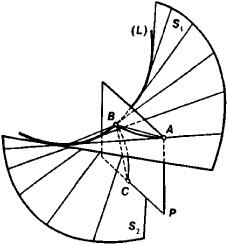# Ruled Surface

(redirected from Doubly ruled)
Also found in: Dictionary.

## ruled surface

[′rüld ′sər·fəs]
(mathematics)
A surface that can be generated by the motion of a straight line.

## Ruled Surface

a surface that can be thought of as a one-parameter family of lines; it may be generated by moving a line (the generatrix) along some curve (the directrix). Ruled surfaces are divided into developable surfaces and nondevelopable surfaces (skew ruled surfaces).

Developable ruled surfaces may be superimposed on a plane by bending. Any developable surface is a cylinder, a cone, or a surface consisting of the tangents to some space curve (L) (Figure 1). This curve is called the edge of regression of the developable surface. If a plane P intersects the edge of regression (L) at a point B, then its intersection with the surface is a curve ABC with cusp B. The edge of regression is a singular curve of a developable surface along which its two nappes S1 and S2 touch.Figure 1

Developable surfaces are also characterized by the invariance of the plane tangent to them at the points of a given generatrix. It follows that the set of tangent planes of a developable ruled surface is a one-parameter family. In other words, a developable ruled surface is the envelope of a one-parameter family of planes.

For a nondevelopable ruled surface the tangent planes are different at different points of a given generatrix. When the point of tangency moves along the generatrix, the tangent plane rotates about the generatrix. A full rotation of the tangent plane, corresponding to a traversal of the entire generatrix by the point of tangency, is equal to 180°. Every generatrix has a point such that for each of the two parts into which it divides the generatrix a full rotation of the tangent plane is equal to 90°. This point (point 0 in Figure 2) is called the center of the generatrix. The tangent of the angle between the planes tangent to the surface at theFigure 2

center 0 and at some other point 0’; of the same generatrix is proportional to the distance 00’. The proportionality factor is called the distribution parameter of the ruled surface. The absolute value of the total curvature of a ruled surface reaches its maximum value on a given generatrix at its center and decreases as we move away from the center along the generatrix. The locus of the centers of generatrices is called the curve of striction. For example, for a helicoid—a ruled surface described by the uniform spiral motion of a line about some axis (which the moving line intersects at a right angle)—the axis (AB in Figure 2) is the curve of striction. Quadric ruled surfaces—the hyperbolic paraboloid, the hyperboloid of one sheet—have two different systems of rectilinear generatrices (the radio tower of V. G. Shukhov’s system, which is located at Shabolovka in Moscow, was constructed from hyperboloids of one sheet). Quadric ruled surfaces are the only doubly ruled surfaces.

If two ruled surfaces can be rolled out on each other, then it is possible to roll one along the other in such a way that they will have a common generatrix. The application of ruled surfaces in the theory of mechanisms is based on this fact.

### REFERENCES

Finikov, S. P. Teoriia poverkhnostei. Moscow-Leningrad, 1934. Pogorelov, A. V. Differentsial’ naia geometriia, 5th ed. Moscow, 1969.

E. G. POZNIAK

Site: Follow: Share:
Open / Close